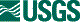## ISIS 2ISIS Application Documentation

# phohillier

Printer Friendly View | TOC | Home

Apply Hillier photometric correction to multiband cubes

 Overview Parameters

## Description

phohillier implements a photometric correction based upon a paper titled "Multispectral Photometry of the Moon and Absolute Calibration of Clementine UV/Vis Camera" by Hillier, Burratti and Hill, published in Icarus 141, 205-225 (1999). The equation for I/F photometric correction from this source is:

```      I/F = (mu0/(mu+mu0)*F(phase)

where:
mu0 = cos(incidence)
mu   = cos(emission)
F(phase) = b0*e(-b1*phase) + a0 + a1*phase + a2*phase^2 +
a3*phase^3 + a4*phase^4
```

The equation described there utilizes the Lommel-Seeliger function to account for scattering dependance on incidence and emission angles. Lunar Reflectance approximately follows this function and this is the dependence expected for signly scattered light. Thus is should be a good approximation for dark objects such as the Moon where singly scattered light dominates reflectance. The phase function is a fourth order polynomial with an exponential term to better account for opposition surge.

This application provides features that allow multiband cubes to be photometrically corrected with a properly formatted PVL input file much like that of ISIS program photomet. This application restricts much of the options available to the more sophiscated photomet application. Below is an example input parameter file for this application:

```Object = NormalizationModel
Group = Algorithm
Name = Albedo
PhotoModel = Hillier
Incref=30.0
Emaref=0.0
Pharef=30.0
EndGroup
EndObject

Object = PhotometricModel
HillierUnits = Degrees
Group = Algorithm
Name = Hillier
FilterName = "Filter1"
BandBinCenter = 100.1
B0 = 0.0432753
B1 = 0.0644091
A0 = -0.0207532
A1 = 0.00165219
A2 = -3.94007e-05
A3  = 4.19325e-07
A4 = -1.69163e-09
EndGroup

Group = Algorithm
Name = Hillier
FilterName = "Filter2"
BandBinCenter = 112.5
B0 = 0.0332283
B1 = 0.00667452
A0 = -0.0258405
A1 = -9.04379e-05
A2 = 7.59709e-06
A3 = -1.06395e-07
A4 = 5.18268e-10
EndGroup

Group = Algorithm
Name = Hillier
FilterName = "Filter8"
BandBinCenter = 545.3
BandBinCenterTolerance = 1.0E-2
B0 = 0.0347020
B1 = 0.0211712
A0 = -0.0244440
A1 = 0.000388924
A2 = 4.72860e-07
A3 = -5.00731e-08
A4 = 3.07309e-10
EndGroup
EndObject
```

The NormalizationModel object will (currently) always apply an Albedo normalization. The value of the Name parameter is ignored here. Also ignored is the value if PhotoModel as it is always "Hillier". The Incref, Emaref and Pharef are the incidence, emission and phase angles to be used as the photometric standard. It will be used to normalize the photometric correction parameter to these angles. The equation used to create the photometrically corrected I/F dn is:

```          odn = idn * (phostd  / ph)

where phostd is the Hillier photometry at the given Incref,
Emaref and Pharef angles
ph        is the photometric correction for the
incidence, emission and phase at each pixel
```

In the above example, parameters B0-B1 and A0-A4 are the parameters for the Hillier equation. Additional parameters area needed in order to apply a specific set of parameters in an Algorithm group to selected bands. The set of parameters, B0-B1, A0-A4, within an Algorithm group are applied to a band if (ABS(Center-BandBinCenter) <= ABS(BandBinCenterTolerance)). The "Center" parameter in the above equality comes from the Center keyword in the BandBin group of the input cube file specified in the FROM parameter. This keyword must exist in the input cube or an error is generated and the program aborts. BandBinCenter and BandBinCenterTolerance are contained in each Algorithm group. Only BandBinCenter is required. If BandBinCenterTolerance is not present in an Algorithm group a value of 1.0E-6 is used. All input bands in the FROM file must be matched to at least one of the Algorithm parameters otherwise an error is generated and the application is aborted.

The parameter HillierUnits is provided to specify if the phase angle is in units of degrees or radians. It does not have to exist in any group or even in the top Object section. If it does not exist, "Radians" is the default.

An additional feature of the PVL structure is that any keyword that exists in the Object section of the PhotometricModel Object is propagated to each Algorithm group when it is read in unless the keyword already exists in the Algorithm group. If a keyword exists in both the PhotometricModel object and an Algorithm group, the keyword in the Algorithm group has precedence.

Below is an example of a small PVL file that will process all bands that have a center wavelength of 100.0 to 900.0 (units do not matter as long as the PVL ALgorithm groups contain the same units as the BandBin Center keyword in the input cube file). The units of phase is provided in Radians for this particular example and is overridden from the keyword in the PhotometricModel object:

```Object = NormalizationModel
Group = Algorithm
Name = Albedo
PhotoModel = Hillier
Incref=30.0
Emaref=0.0
Pharef=30.0
EndGroup
EndObject

Object = PhotometricModel
HillierUnits = Degrees
Group = Algorithm
Name = Hillier
FilterName = "AllFilters"
BandBinCenter = 500.0
BandBinCenterTolerance = 400.0
B0 = 0.0347020
B1 = 0.0211712
A0 = -0.0244440
A1 = 0.000388924
A2 = 4.72860e-07
A3 = -5.00731e-08
A4 = 3.07309e-10
EndGroup
EndObject
```

Additional consequences of the photometric correction processing is any incidence angle greater than i90 degrees is set to the ISIS special Null pixel value. And, of course, any ISIS special pixel encountered on input is propagated to the output TO file without modification.

## History

 Kris Becker 2010-02-21 Original version.

## Parameter Groups

Name Description
FROM Input cube
TO Output cube
PHOPAR Pvl file

### Files: FROM

#### Description

Use this parameter to select the input filename.

 Type cube input *.cub

### Files: TO

#### Description

This file will contain the photometrically corrected image data after being corrected by with Hillier algorithm.

 Type cube output real

### Files: PHOPAR

#### Description

This file will contain the parameters B0-B1, A0-A4 to use when applying the Hellier photometric correction. See the main program documentation for a full description.

 Type filename input *.pvl